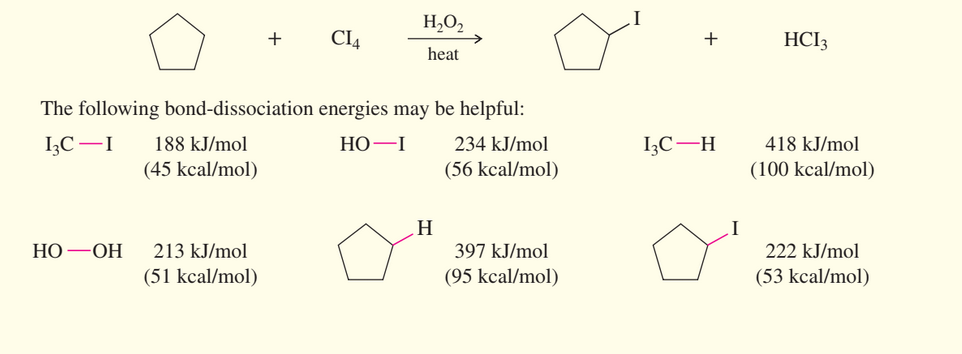×
Get Full Access to Organic Chemistry - 8 Edition - Chapter 4 - Problem 57sp
Get Full Access to Organic Chemistry - 8 Edition - Chapter 4 - Problem 57sp

×

# Iodination of alkanes using iodine (I2) is usually anISBN: 9780321768414 33

## Solution for problem 57SP Chapter 4

Organic Chemistry | 8th Edition

• Textbook Solutions
• 2901 Step-by-step solutions solved by professors and subject experts
• Get 24/7 help from StudySoup virtual teaching assistantsOrganic Chemistry | 8th Edition

4 5 1 342 Reviews
14
5
Problem 57SP

Iodination of alkanes using iodine $$\left(\mathrm{I}_{2}\right)$$ is usually an unfavorable reaction. (See Problem 4-17, for example.) Tetraiodomethane $$\left(\mathrm{Cl}_{4}\right)$$ can be used as the iodine source for iodination, in the presence of a free-radical initiator such as hydrogen peroxide. Propose a mechanism (involving mildly exothermic propagation steps) for the following proposed reaction. Calculate the value of  $$\Delta H$$ for each of the steps in your proposed mechanism.Equation Transcription:Text Transcription:

(I2)

(Cl4)

\Delta H

CI4

H2O2

HCI3

I3C-I

HO-I

Step-by-Step Solution:

Solution  57SP

Here, we are going to calculate the value offor each of the steps in your proposed mechanism.Step 1:

MechanismHere, Bond dissociation energy of bonds broken :

HO-OH = 213 kJ/mol

I-CI3 = 188 kJ/mol

Bond dissociation energy for bonds formed :

HO-I = 234 kJ/molo = [Bond dissociation energy for bonds broken] -[Bond dissociation energy for bonds formed]

= +188 kJ/mol - 234 kJ/mol = -46.0 kJ/mol

Step 2 of 3

Step 3 of 3

##### ISBN: 9780321768414

Since the solution to 57SP from 4 chapter was answered, more than 1511 students have viewed the full step-by-step answer. The answer to “?Iodination of alkanes using iodine $$\left(\mathrm{I}_{2}\right)$$ is usually an unfavorable reaction. (See 4-17, for example.) Tetraiodomethane $$\left(\mathrm{Cl}_{4}\right)$$ can be used as the iodine source for iodination, in the presence of a free-radical initiator such as hydrogen peroxide. Propose a mechanism (involving mildly exothermic propagation steps) for the following proposed reaction. Calculate the value of $$\Delta H$$ for each of the steps in your proposed mechanism. Equation Transcription:Text Transcription:(I2)(Cl4)\Delta HCI4H2O2HCI3I3C-IHO-I” is broken down into a number of easy to follow steps, and 69 words. Organic Chemistry was written by and is associated to the ISBN: 9780321768414. This textbook survival guide was created for the textbook: Organic Chemistry, edition: 8. The full step-by-step solution to problem: 57SP from chapter: 4 was answered by , our top Chemistry solution expert on 05/06/17, 06:41PM. This full solution covers the following key subjects: Iodination, iodine, proposed, steps, reaction. This expansive textbook survival guide covers 25 chapters, and 1336 solutions.

## Discover and learn what students are asking

Calculus: Early Transcendental Functions : Linear Models and Rates of Change
?In Exercises 5-10, plot the pair of points and find the slope of the line passing through them. (1,1), (-2,7)

Statistics: Informed Decisions Using Data : Data Collection
?What is meant by the process of statistics?

Chemistry: The Central Science : Atoms, Molecules, and Ions
?Fill in the gaps in the following table:

Unlock Textbook Solution